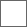• 22回复贴，共1

### 我也记录下收入和支出，看看到年底能存多少钱，目标是存两万。

100+100+10+10=120

120+15=135155+60=215

379+81=460

2200+460=2660

2660+30=2690

2690+260+200+330=3480

3480+21=3501

3501+38=3539

3737+100=3837

4084+174=4258

14+400=414
4528+414=49424942+78+160=5180

5180+45=52255225+15=5240

5240+100+56=5396+2=5398

26+55+30+172+264=547
5411+547=5958

152+600=752